# Push-pull HF preamplifier with 2x2N5109

I have built the push-pull preamplifier for the HF bands described some years ago in the Mar/Apr 2003 issue of QEX (article is also available on the author's website here); its performances are quite good and the measurements I have done practically confirms the specifications stated in the article.

The schematic diagram, drawn with Qucs is shown below:and here is how I built it: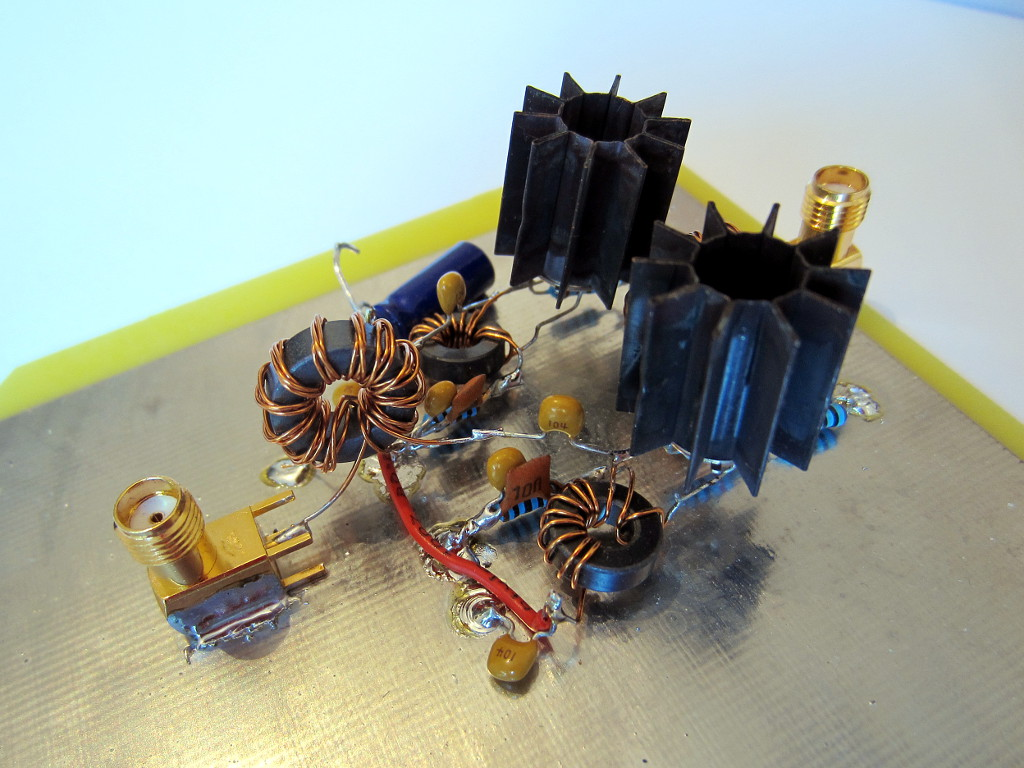The measured small-signal S-parameters together with the simulated ones; differences at high frequencies are likely due to inaccurate transformer and parasitics modeling.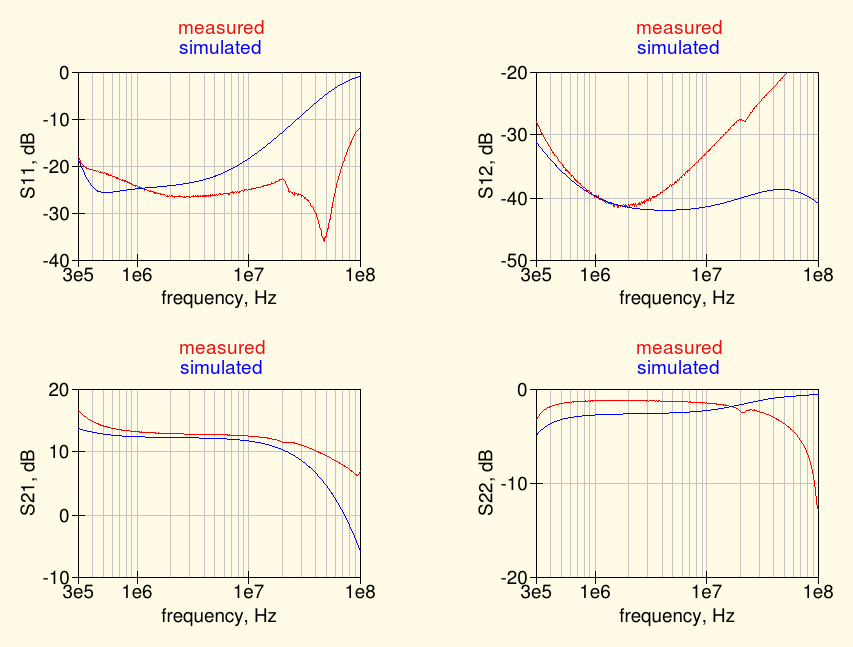.

the gain at 10 MHz is about 12.5 dB, peaking at low frequency due to the interaction between the input transformer inductance and the coupling capacitors; reducing the value of the two 15 nF capacitors should help in flattening the gain at low frequency. The output return loss is quite high, since the output impedance is much lower than 50 ohm, a known issue of this kind of preamplifier.

The fundamental and harmonics output levels versus input power, with a 10 MHz sine-wave input, are shown in the graph below:RF output and DC current drawn from the supply versus input power (10 MHz sine wave) are shown in the following graph: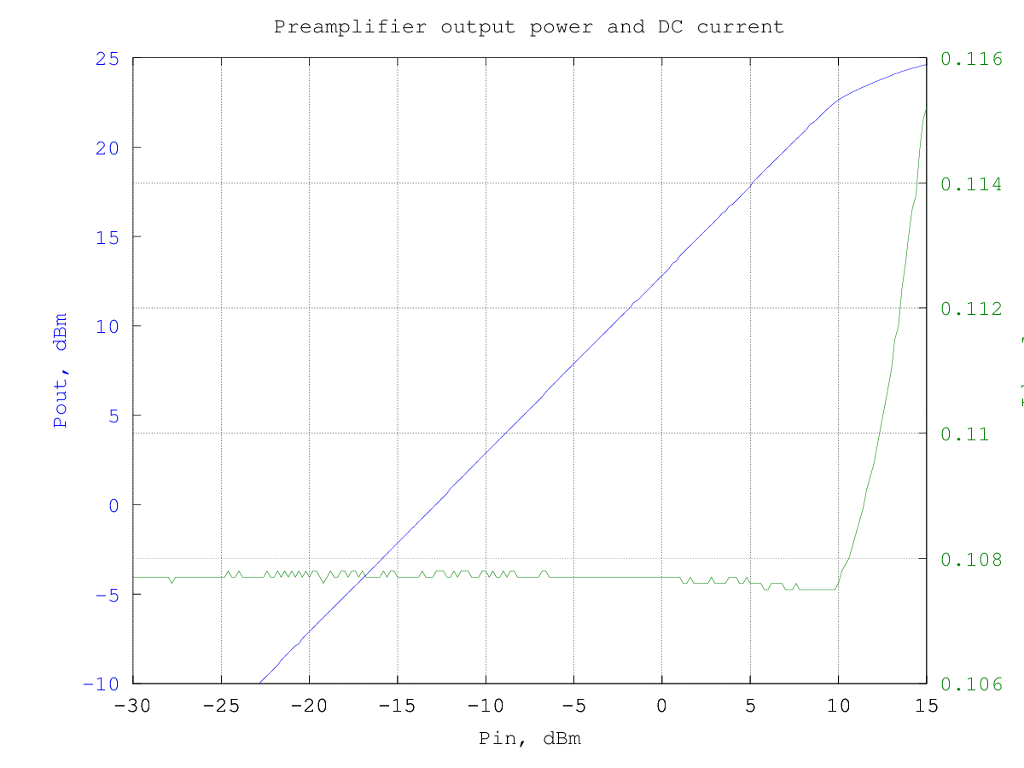The intermodulation products (3rd, 5th and 7th order) amplitude w.r.t the output power is shown in the graph below (note that the output power shown is per-tone):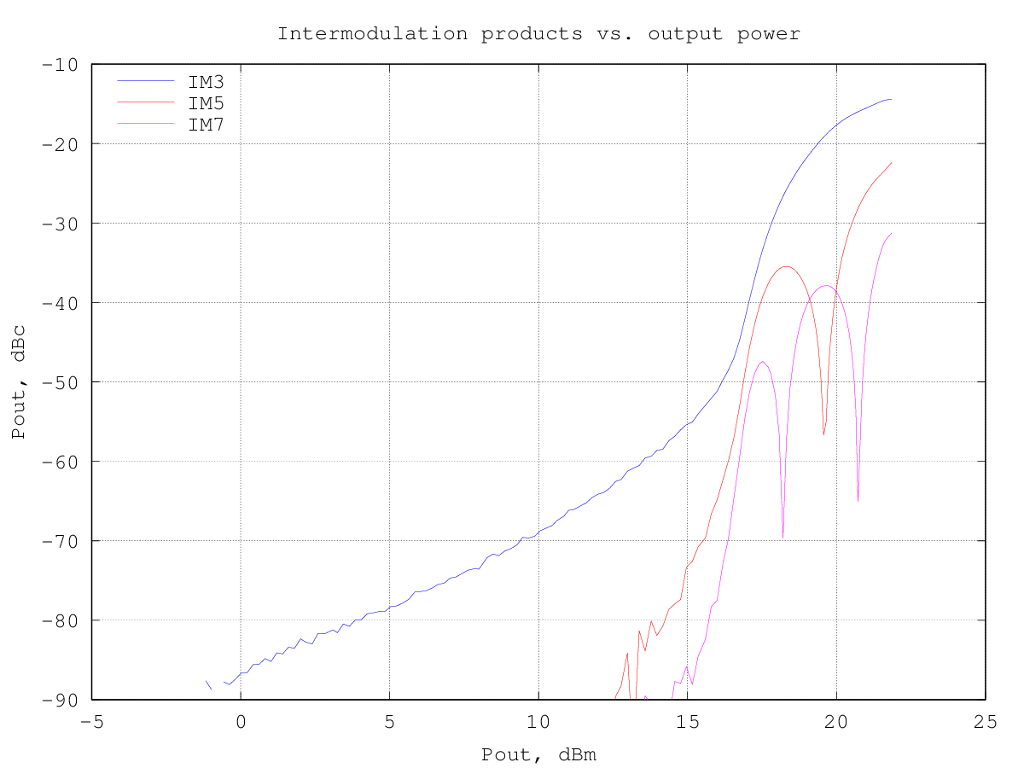By plotting the third-order intermodulation products power vs. the input power we can find the intercept point by extrapolating the results. The amplifier third-order intermodulation products follow the theoretical 3:1 slope w.r.t. the input power up to about 0dBm input, as can be seen from the graph below:The input IP3 turns out to be 31.4 dBm and the corresponding output IP3 is 44.3 dBm.

The graph below shows the relative amplitude of the IMD products (separately for upper and lower products) for a two-tone test with varying tone spacing (the output power is 13.6 dBm (22.9 mW) per tone)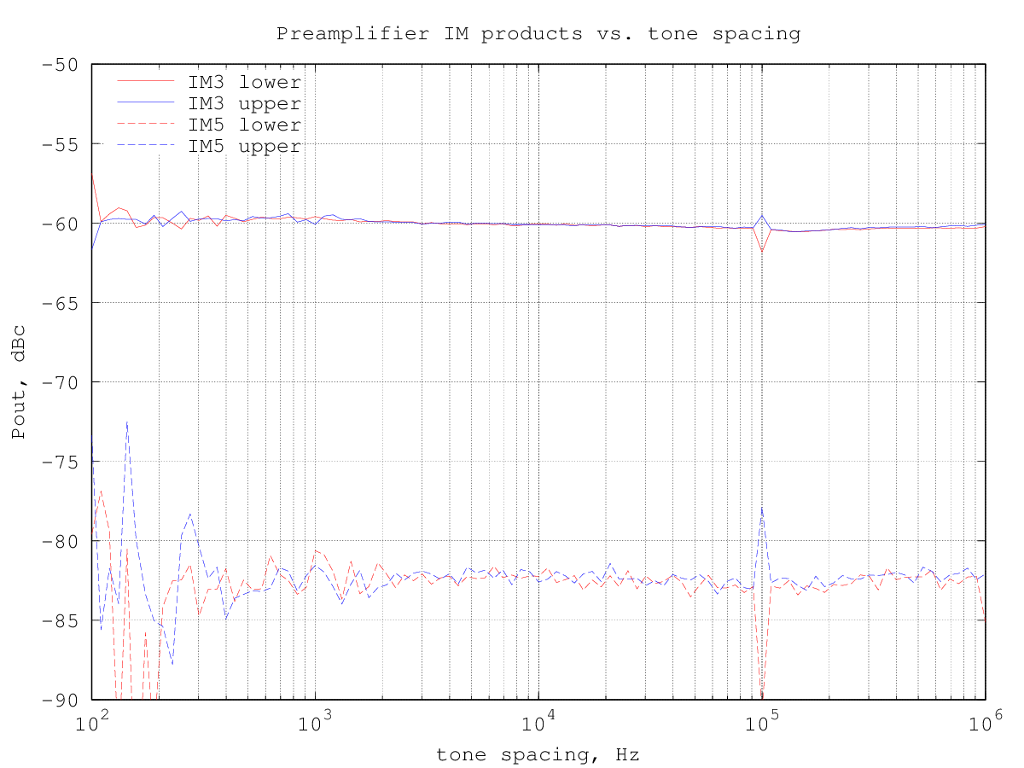.

the IMD products power is practically independent from the tone spacing.